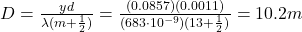## Using a 683 nm wavelength laser, you form the diffraction pattern of a 1.1 mm wide slit on a screen. You measure on the screen tha

Question

Using a 683 nm wavelength laser, you form the diffraction pattern of a 1.1 mm wide slit on a screen. You measure on the screen that the 13 th dark fringe is 8.57 cm away from the center of the central maximum. How far is the screen located from the slit

in progress 0
2 months 2021-08-01T04:34:11+00:00 1 Answers 3 views 0

10.2 m

Explanation:

The position of the dark fringes (destructive interference) formed on a distant screen in the interference pattern produced by diffraction from a single slit are given by the formula: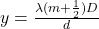where

y is the position of the m-th minimum

m is the order of the minimum

D is the distance of the screen from the slit

d is the width of the slit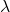is the wavelength of the light used

In this problem we have: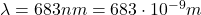is the wavelength of the light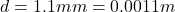is the width of the slit

m = 13 is the order of the minimum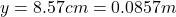is the distance of the 13th dark fringe from the central maximum

Solving for D, we find the distance of the screen from the slit: